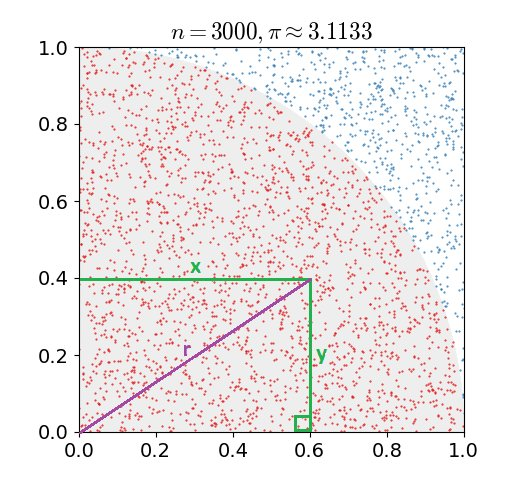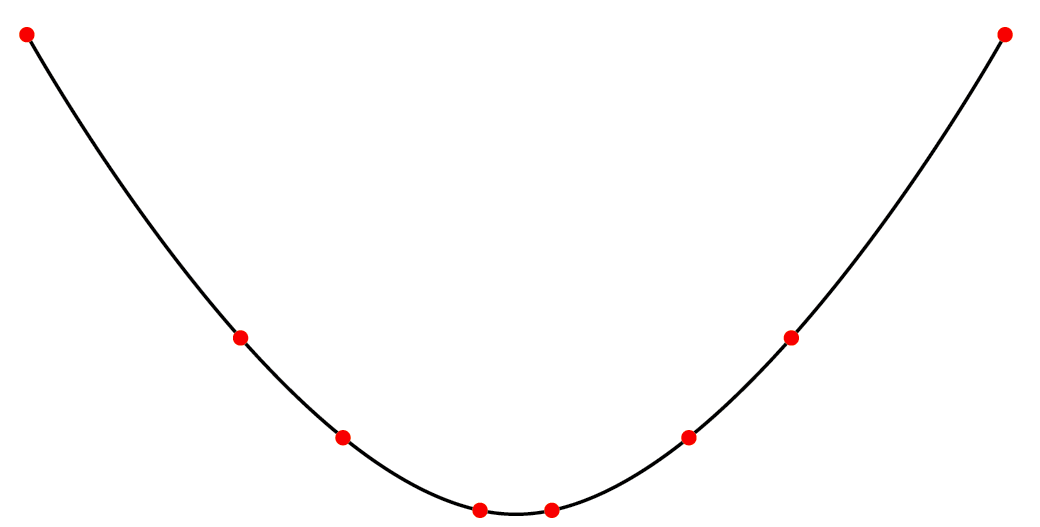# Whose spline is it anyway?

By Laura Sach. Posted

### How mathematical terminology can obscure otherwise straightforward concepts in Computer Science

I didn’t take A-level Mathematics, but I really should have done. I’m sorry Mrs Britton, you were right. Starting my Computer Science degree course would have felt less like being asked to walk on stage for the first time and sing an opera to a live audience if I had been better prepared with some mathematical hand-holding beforehand.

It is highly likely that you will remember the first time you tried learning something in the field of computer science and got completely lost. One of my first-year university lecturers insisted on writing elaborate and lengthy Boolean equations on the blackboard, before proclaiming that the proof I had been eagerly waiting for was ‘trivial’. I still remember the panic that I should be able to prove it, and the feeling of powerlessness and inadequacy that I didn’t know what it was that I didn’t know – I didn’t even know where to start looking.

When I became a teacher, I vowed to never knowingly make any of my students feel this way about a computer science topic in the classroom. I have been forced to revisit some topics I initially disliked as they are on the A-level specification and – gulp – now I’m the teacher. Every time I have gone to my trusty textbook only to come away with the revelation of, “oh it’s just that”. The thing I was supposed to be learning was indeed relatively easy to grasp, provided you had a friendly and supportive explanation to help you through the mental steps of the initial learning experience.

### Terminology and symbols

Mathematics comes with some pretty heavy-duty terminology, which makes it much more difficult for people new to a concept to access or learn about it. Bear with me here, because I’m going to show you how this works. Imagine I’ve set you this assignment:

#### “Write a program using a Monte Carlo method for estimating the value of π.”

In one sentence I’ve done two things that (depending on your mathematical background) might potentially cause you considerable anxiety. Do you know what a Monte Carlo method is? Should you? Are you already behind if you don’t? I’ve also included the Greek for pi (π), which you may well be familiar with. But, what if it was a different Greek letter like Σ or λ? Would you even know how to say that letter out loud? Would you want to ask a question in a lesson about something you weren’t even sure how to pronounce?

Here’s an explanation of how you would actually go about using this method:

• Imagine a quarter circle with radius 1 inside a 1×1 square

• Generate lots of random points that fall inside the square, like darts thrown blindfold at a dartboard

• You can estimate pi by counting how many points fall inside the circle, compared to the total number of points

A Monte Carlo method is a method that uses repeated random sampling. Here’s a picture of what generating those points and drawing them on a graph might look like.Do you remember Pythagoras’ theorem, which is used for calculating the length of the sides of a right angled triangle?

a² + b² = c²

• The indicated point in the diagram has the coordinates x = 0.6 and y = 0.4

• We need to find the distance of this example point from 0,0 to check whether or not it is inside the circle. We’ll call this distance r for radius (see the purple line)

• Plug the values of x and y into Pythagoras’ theorem to calculate the radius squared:

• = 0.6² + 0.4² = 0.36 + 0.16 = 0.52

• Finally, take the square root to get the radius. If the result is less than 1, the point is inside the circle. If it is 1 or greater, the point is outside the circle.

Here’s the program for generating 100 points and calculating how many are inside the circle:

``````inside = 0
n = 100

FOR i FROM 0 TO n
x = (RANDOM number between 0 and 1)
y = (RANDOM number between 0 and 1)
result = SQUARE_ROOT(x*x + y*y)
IF result <= 1
inside = inside + 1
ENDIF
ENDFOR``````

### Making maths accessible

I should be very clear here that I am not saying that every mathematical concept is secretly very easy to learn and has just been explained to you badly, because that’s not the case. Hard things are hard. However, I think there are some ways that you can make topics which include some mathematical background knowledge much more palatable to learners who are less confident. Here are some things I have tried which work for me:

Avoid using words and phrases like ‘simply’, ‘just’ and ‘trivial’. Your trivial might be someone else’s nemesis.

Always call out any background knowledge assumptions in a friendly way. In the topic above I reminded you of Pythagoras’ theorem, but I tried hard to make sure you wouldn’t feel belittled if you couldn’t remember it or its application.

Don’t shortcut bits you think are easy or obvious. If you look at the full resource for estimating the value of pi (rpf.io/octapi-pi), taking the square root of r is skipped entirely in the code. This is possible because if a number is less than 1, the square root of that number is also guaranteed to be less than 1 and so it saves a computational operation. However, if you don’t know or don’t remember this fact, you might be thrown off. I also have not explained in the article why this method works to estimate pi, but the full explanation is in the resource.

### Maths in computer science

Lots of computer science topics studied at school have a natural crossover with mathematics. Here are a few examples:

• Variables – just like in mathematics where students have used x and y (but be careful not to introduce misconceptions)

• Functions – for example in mathematics, f(x) = 2x is a function, it works in exactly the same way as functions we write in a program

• Video game graphics – trigonometry and vectors are used to calculate the movements of a character model. The ‘spline’ referred to in the title is a curve connecting points on a graph.

• Encryption – your data is protected by a really hard maths problem (finding prime factors of a very large number)

• Machine learning – many modern methods are based on differentiation (and more vectors!)## Print

Free - UK only

If you’re a UK-based teacher, volunteer, librarian or something in between, we'll send each issue free to your door.

## Digital

Free

Just want to read the free PDF? Get each new issue delivered straight to your inbox. No fuss and no spam.